# Travelling Salesman Problem using Hungarian method

A travelling salesman plans to visit N cities in such a way that he visits each city exactly once and return to the city from where he started. The distance between City i and City j is Cij. Find the shortest tour he can take.

Note: Travelling from City i to City i is not possible and Cij may not be equal to Cji.

Examples:

Input: See the routes in the image.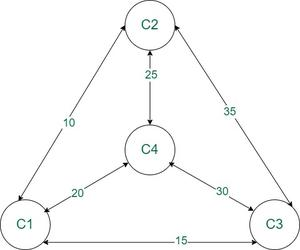Output: 80
Explanation: The shortest route can be achieved following the path C1 -> C2 -> C4 -> C3 -> C1
So the route length is 10 + 25 + 30 + 15 = 80.

Input: See the routes in the image.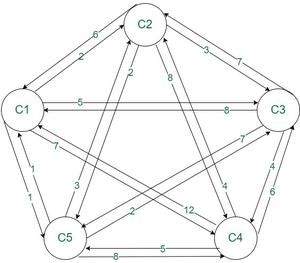Output: 15
Explanation: The shortest route is C1 -> C2 -> C3 -> C4 -> C5 -> C1. So the path length is 2 + 3 + 4 + 5 + 1 = 15.

Intuition: Suppose there are 3 cities namely city 1 ,city 2 , and city 3.  All the possible combinations to visit the cities are

1->2->3->1       (1->2, 2->3, 3->1)
1->3->2->1       (1->3, 3->2, 2->1)
2->1->3->2       (2->1, 1->3, 3->2)
2->3->1->2       (2->3, 3->1, 1->2)
3->1->2->3       (3->1, 1->2, 2->3)
3->2->1->3       (3->2, 2->1,1->3)

So, the cities can be visited in 6 different ways but if observed carefully it can be seen that tour 1st, 4th and 5th are equivalent and tour 2nd, 3rd and 6th are equivalent. Therefore, different combinations possible are actually 2 and those are

1->2->3->1
1->3->2->1

Hence, If there are N cities to visit then there can be (N-1)! ways to travel to each city exactly once and return to the starting city. This type of problem can be solved by the Hungarian method, branch and bound method, penalty method, and nearest neighbor method. We will see how to solve this type of problem using Hungarian method.

Approach: Mentioned below are the steps to follow to solve the problem using Hungarian method.
Consider the example shown in the image: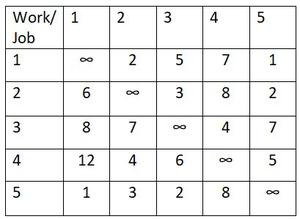Follow the illustrations of solution of the above example for better understanding.

Step 1: Locate the smallest cost elements in each row of the cost matrix. Subtract this element from every other element of the corresponding row. As a result, there is at least one zero in each row of the reduced cost matrix

Illustration:

1. Subtracting 1st row with the minimum value 1.
2. Subtracting 2nd row with the minimum value 2.
3. Subtracting 3rd row with the minimum value 4.
4. Subtracting 4th row with the minimum value 4.
5. Subtracting 5th row with the minimum value 1.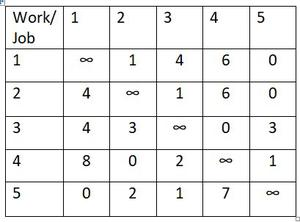Step 2: Similarly, locate the smallest element of each column In the reduced cost matrix obtained and subtract that from each element of the corresponding column. As a result, there should be at least one zero in each row and columns of the second reduced cost matrix.

Illustration:

1. Subtracting 1st column with the minimum value 0.
2. Subtracting 2nd column with the minimum value 0.
3. Subtracting 3rd column with the minimum value 1.
4. Subtracting 4th column with the minimum value 0.
5. Subtracting 5th column with the minimum value 0.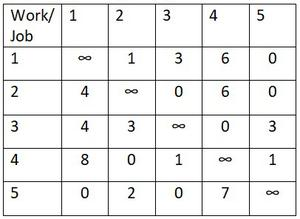Step 3: Make assignment in the matrix as follows.

1. Move row by row until a row with single zero is found .
2. Assign the cell containing the zero and cross off all other zeros in its column.
3. Continue this process until all the rows with single zero is assigned.
4. Repeat the procedure for each column.
5. If a row and ( or ) a column has two or more zeros and one cannot be chosen by inspection then assign arbitrary any one of these zeros and cross off all other zeros of that row / column.

Illustration:

Row wise cell (1,5) is assigned. So, column wise cell (2,5) is crossed off.
Row wise cell (2,3) is assigned. So, column wise cell (5,3) is crossed off.
Row wise cell (3,4) is assigned.
Row wise cell (4,2) is assigned.
Row wise cell (5,1) is assigned.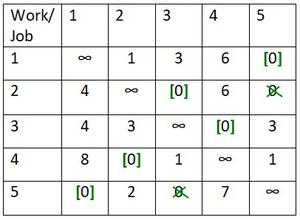Here, the number of assignment is equal to the number of cities and the tour is 1->5->1 and 2->3->4->2 which means salesman will travel from city 1 to city 5 and return to city 1 and then again start from city , travel to city 3, city 4 and return to city 2. Therefore, we are getting two cycle and hence it is not the solution.

Step 4: The next best solution can be obtained by bringing the minimum non-zero element and repeat the step 2 and step 3

Illustration: In this case, minimum non-zero element is 1.

Row wise cell (3,4) is assigned.
Row wise cell (1,2) is assigned. So, column wise cell (4,2) is crossed off and row wise cell (1,5) is crossed off.
Row wise cell (2,5) is assigned. So, row wise cell (2,3) is crossed off and column wise cell (4,5) is crossed off.
Row wise cell (4,3) is assigned. So, column wise cell (5,3) is crossed off.
Row wise cell (5,1) is assigned.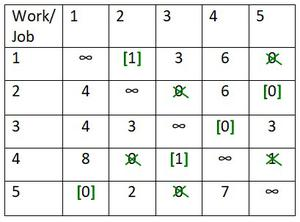Now, the sequence is 1->2->5->1 and 3->4->3. Again, we are getting two cycle therefore this is also not the solution.

Step 5:  Make different possible assignment

Illustration:

Row wise cell (3,4) is assigned.
Row wise cell (1,2) is assigned. So, column wise cell (4,2) is crossed off and row wise cell (1,5) is crossed off.
Row wise cell (2,3) is assigned. So, column wise cell (4,3) and (5,3) is crossed off and row wise cell (2,5) is crossed off.
Row wise cell (4,5) is assigned.
Row wise cell (5,1) is assigned.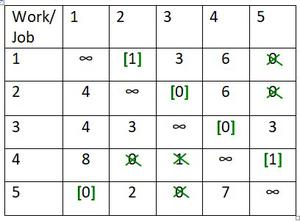The sequence is 1->2->3->4->5->1. So, this solution is optimal and total distance of this tour is

Cell12 + Cell23 + Cell34 + Cell45 + Cell51 = (2+3+4+5+1) =15.

Feeling lost in the world of random DSA topics, wasting time without progress? It's time for a change! Join our DSA course, where we'll guide you on an exciting journey to master DSA efficiently and on schedule.
Ready to dive in? Explore our Free Demo Content and join our DSA course, trusted by over 100,000 geeks!

Previous
Next